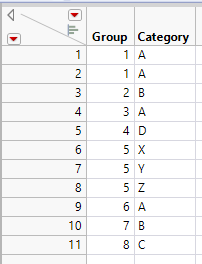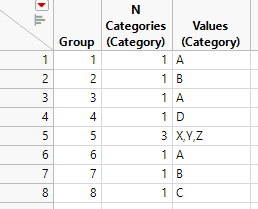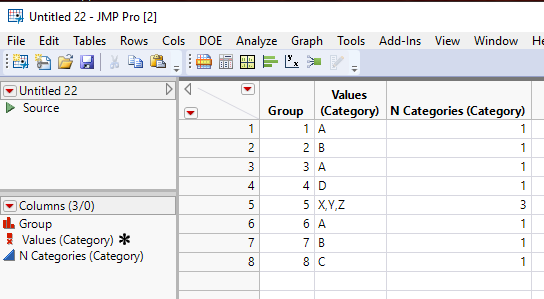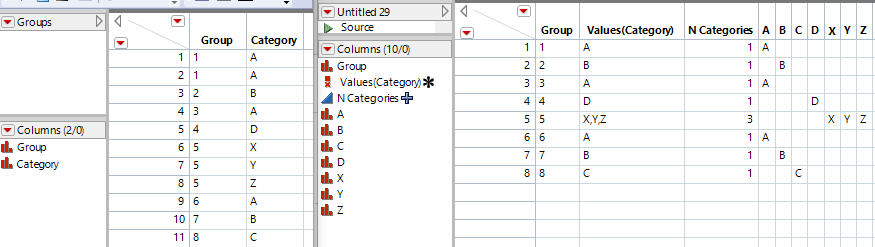Choose Language Hide Translation Bar
Highlighted

## Summary of categorical levels

I want to get a summary of the values of a column, using another as a "By" group.  It's like what Summary() does with N Categories, but I want a list of the actual values, not just the number of them.  For example take this table:I want something like:I'm thinking I could do something with using the 'Category' as a subgroup in Summary(), then Recode and Combine Columns()?  Any better or more clever ideas?  I poked around the community a bit and couldn't find anything analgous (suprisingly?)  Maybe a place for use of Associative Arrays?  This example table is tiny, the table I will be running this on could be a few million rows with hundreds of "levels" for the Group column.

3 ACCEPTED SOLUTIONS

Accepted Solutions
Highlighted

## Re: Summary of categorical levels

I think the way to start is to do a split on the data table, and then to work from there.  Below is a simple script that I wrote to get you the results you want.  If your need is to do this interactively, just follow the steps in the script``````Names Default To Here( 1 );

// Create the beginning table
dt = New Table( "Example",
New Column( "Group",
Numeric,
"Continuous",
Format( "Best", 12 ),
Set Values( [1, 1, 2, 3, 4, 5, 5, 5, 6, 7, 8] )
),
New Column( "Category",
Character,
"Nominal",
Set Values( {"A", "A", "B", "A", "D", "X", "Y", "Z", "A", "B", "C"} )
)
);

// Change the group column to character so
// split columns are blanks and not missing values
dt:Group << data type( character );

// Split the columns
dtSplit = dt << Split( Split By( :Category ), Split( :Group ), Group( :Group ), Sort by Column Property );

// Change the data type back
dt:Group << data type( numeric );
dtSplit:Group << data type( numeric );

// Convert all of the non blank entries to the value of the column name
For( i = 2, i <= N Cols( dtSplit ), i++,
theRows = dtSplit << get rows where( As Column( dtsplit, i ) != "" );
If( N Rows( theRows ) > 0,
Column( dtSplit, i )[theRows] = Column( dtsplit, i ) << get name
);
);

// get all of the character column names
namesList = dtSplit << get column names( character, string );

// Create the combined column
dtSplit << Combine Columns(
delimiter( "," ),
Columns( :a, :b, :c, :d, :X, :y, :z ),
Column Name( "Values (Category)" )
);

// Count the number of combined values
dtSplit << New Column( "N Categories (Category)",
Numeric,
"Continuous",
Format( "Best", 12 ),
Formula(
count = 1;
While( Word( count, :Name( "Values (Category)" ), "," ) != "", count++ );
count = count - 1;
)
);

// get rid of the formula
dtSplit:Name("N Categories (Category)") << delete property(formula);

// delete the unnecessary columns
dtSplit << delete columns( namesList );``````

Jim
Highlighted

## Re: Summary of categorical levels

I see now Jim has already presented a solution.

Here's a different approach using Tabulate and Combine Columns (Multiple Response Column) that hopefully works also on the full data set.

``````// Groups is the example table with 2 nominal columns
DT_GroupCategory = Data Table( "Groups" );

// creates a cross table
Tab_DT_GroupCategory = DT_GroupCategory << Tabulate(
Column Table( Grouping Columns( :Category ) ),
Row Table( Grouping Columns( :Group ) )
)
);

// make data table from it
DT_GroupCategory_CrossTab = Tab_DT_GroupCategory << Make Into Data Table;
DT_GroupCategory_CrossTab << Add Multiple Columns( "N Categories", 1, after( 1 ), Numeric );
Names_DT_GroupCategory_CrossTab = DT_GroupCategory_CrossTab << Get Column Names;

// replace 0 by missing and everything else by column name (probably not the most efficient way to do this)
For( i=3, i<=N Items( Names_DT_GroupCategory_CrossTab ), i++,
Column( DT_GroupCategory_CrossTab, i ) << Data Type( "Character" );
For( j=1, j<=N Rows( DT_GroupCategory_CrossTab ), j++,
If( Column( DT_GroupCategory_CrossTab, i )[j] == "0",
Column( DT_GroupCategory_CrossTab, i )[j] = "",
Column( DT_GroupCategory_CrossTab, i )[j] = Char( Names_DT_GroupCategory_CrossTab[i] );
)
)
);

// make multiple response column with Cols -> Utilities -> Combine Columns
DT_GroupCategory_CrossTab << Combine Columns(
delimiter( "," ),
Columns( 2::N Items( Names_DT_GroupCategory_CrossTab ) ),
Selected Columns are Indicator Columns( 0 ),
Column Name( "Values(Category)" )
);

// add formula to get N Categories. +1 is added for one less comma, then div by 2 to get number of categories
Column( DT_GroupCategory_CrossTab, "N Categories" ) << Formula( (Length( :Name( "Values(Category)" ) ) + 1) / 2 );

Tab_DT_GroupCategory << Close Window;``````Highlighted

## Re: Summary of categorical levels

Thanks to both for the solutions - I ended up doing something with an associative array.  I'll dump that in here with the hopes that one of these three approaches works for the next person trying to do this:

``````Names Default To Here( 1 );

// Create the beginning table
dt = New Table( "Example",
New Column( "Group",
Numeric,
"Continuous",
Format( "Best", 12 ),
Set Values( [1, 1, 2, 3, 4, 5, 5, 5, 6, 7, 8] )
),
New Column( "Category",
Character,
"Nominal",
Set Values( {"A", "A", "B", "A", "D", "X", "Y", "Z", "A", "B", "C"} )
)
);

// let Summary do N Categories() for us
dt_sum = dt << Summary( Group( :Group ), N Categories( Category ) );

// the name of the column for which we want a list of the categories
category_col = "Category";
sum_colname = "Value List("|| category_col || ")";

// new col in the summary table to hold the list of values
dt_sum << New Column(sum_colname, Character);

// dump the group col into a matrix for quicker access
sum_groupdat = dt_sum:Group << Get As Matrix();
main_groupdat = dt:Group << Get As Matrix();

For( ri = 1, ri <= N Rows( dt_sum ), ri++,
// rows in main table that match the 'group by' col
inds = Loc( main_groupdat, sum_groupdat[ri] );

tmpaa = Associative Array( Column( dt, category_col )[inds] );
keys = tmpaa << Get Keys();
// if the category_col is numeric, need to convert the numbers to chars!
If( Is Number( keys ),
charkeys = {};
For( ki = 1, ki <= N Items( keys ), ki++,
charkeys[ki] = Char( keys[ki] );
);
,
charkeys = keys
);	// stuff all the items together and dump it into this row
valstr = Concat Items( charkeys, "," );
Column( dt_sum, sum_colname )[ri] = valstr;
);``````

4 REPLIES 4
Highlighted

## Re: Summary of categorical levels

I think the way to start is to do a split on the data table, and then to work from there.  Below is a simple script that I wrote to get you the results you want.  If your need is to do this interactively, just follow the steps in the script``````Names Default To Here( 1 );

// Create the beginning table
dt = New Table( "Example",
New Column( "Group",
Numeric,
"Continuous",
Format( "Best", 12 ),
Set Values( [1, 1, 2, 3, 4, 5, 5, 5, 6, 7, 8] )
),
New Column( "Category",
Character,
"Nominal",
Set Values( {"A", "A", "B", "A", "D", "X", "Y", "Z", "A", "B", "C"} )
)
);

// Change the group column to character so
// split columns are blanks and not missing values
dt:Group << data type( character );

// Split the columns
dtSplit = dt << Split( Split By( :Category ), Split( :Group ), Group( :Group ), Sort by Column Property );

// Change the data type back
dt:Group << data type( numeric );
dtSplit:Group << data type( numeric );

// Convert all of the non blank entries to the value of the column name
For( i = 2, i <= N Cols( dtSplit ), i++,
theRows = dtSplit << get rows where( As Column( dtsplit, i ) != "" );
If( N Rows( theRows ) > 0,
Column( dtSplit, i )[theRows] = Column( dtsplit, i ) << get name
);
);

// get all of the character column names
namesList = dtSplit << get column names( character, string );

// Create the combined column
dtSplit << Combine Columns(
delimiter( "," ),
Columns( :a, :b, :c, :d, :X, :y, :z ),
Column Name( "Values (Category)" )
);

// Count the number of combined values
dtSplit << New Column( "N Categories (Category)",
Numeric,
"Continuous",
Format( "Best", 12 ),
Formula(
count = 1;
While( Word( count, :Name( "Values (Category)" ), "," ) != "", count++ );
count = count - 1;
)
);

// get rid of the formula
dtSplit:Name("N Categories (Category)") << delete property(formula);

// delete the unnecessary columns
dtSplit << delete columns( namesList );``````

Jim
Highlighted

## Re: Summary of categorical levels

I see now Jim has already presented a solution.

Here's a different approach using Tabulate and Combine Columns (Multiple Response Column) that hopefully works also on the full data set.

``````// Groups is the example table with 2 nominal columns
DT_GroupCategory = Data Table( "Groups" );

// creates a cross table
Tab_DT_GroupCategory = DT_GroupCategory << Tabulate(
Column Table( Grouping Columns( :Category ) ),
Row Table( Grouping Columns( :Group ) )
)
);

// make data table from it
DT_GroupCategory_CrossTab = Tab_DT_GroupCategory << Make Into Data Table;
DT_GroupCategory_CrossTab << Add Multiple Columns( "N Categories", 1, after( 1 ), Numeric );
Names_DT_GroupCategory_CrossTab = DT_GroupCategory_CrossTab << Get Column Names;

// replace 0 by missing and everything else by column name (probably not the most efficient way to do this)
For( i=3, i<=N Items( Names_DT_GroupCategory_CrossTab ), i++,
Column( DT_GroupCategory_CrossTab, i ) << Data Type( "Character" );
For( j=1, j<=N Rows( DT_GroupCategory_CrossTab ), j++,
If( Column( DT_GroupCategory_CrossTab, i )[j] == "0",
Column( DT_GroupCategory_CrossTab, i )[j] = "",
Column( DT_GroupCategory_CrossTab, i )[j] = Char( Names_DT_GroupCategory_CrossTab[i] );
)
)
);

// make multiple response column with Cols -> Utilities -> Combine Columns
DT_GroupCategory_CrossTab << Combine Columns(
delimiter( "," ),
Columns( 2::N Items( Names_DT_GroupCategory_CrossTab ) ),
Selected Columns are Indicator Columns( 0 ),
Column Name( "Values(Category)" )
);

// add formula to get N Categories. +1 is added for one less comma, then div by 2 to get number of categories
Column( DT_GroupCategory_CrossTab, "N Categories" ) << Formula( (Length( :Name( "Values(Category)" ) ) + 1) / 2 );

Tab_DT_GroupCategory << Close Window;``````Highlighted

## Re: Summary of categorical levels

Thanks to both for the solutions - I ended up doing something with an associative array.  I'll dump that in here with the hopes that one of these three approaches works for the next person trying to do this:

``````Names Default To Here( 1 );

// Create the beginning table
dt = New Table( "Example",
New Column( "Group",
Numeric,
"Continuous",
Format( "Best", 12 ),
Set Values( [1, 1, 2, 3, 4, 5, 5, 5, 6, 7, 8] )
),
New Column( "Category",
Character,
"Nominal",
Set Values( {"A", "A", "B", "A", "D", "X", "Y", "Z", "A", "B", "C"} )
)
);

// let Summary do N Categories() for us
dt_sum = dt << Summary( Group( :Group ), N Categories( Category ) );

// the name of the column for which we want a list of the categories
category_col = "Category";
sum_colname = "Value List("|| category_col || ")";

// new col in the summary table to hold the list of values
dt_sum << New Column(sum_colname, Character);

// dump the group col into a matrix for quicker access
sum_groupdat = dt_sum:Group << Get As Matrix();
main_groupdat = dt:Group << Get As Matrix();

For( ri = 1, ri <= N Rows( dt_sum ), ri++,
// rows in main table that match the 'group by' col
inds = Loc( main_groupdat, sum_groupdat[ri] );

tmpaa = Associative Array( Column( dt, category_col )[inds] );
keys = tmpaa << Get Keys();
// if the category_col is numeric, need to convert the numbers to chars!
If( Is Number( keys ),
charkeys = {};
For( ki = 1, ki <= N Items( keys ), ki++,
charkeys[ki] = Char( keys[ki] );
);
,
charkeys = keys
);	// stuff all the items together and dump it into this row
valstr = Concat Items( charkeys, "," );
Column( dt_sum, sum_colname )[ri] = valstr;
);``````

Highlighted

## Re: Summary of categorical levels

It would be appropriate for you to mark all of the responses that provided you with a correct answer as a Solution, so that future readers can see the different ways to solve the problem.
Jim
Article Labels

There are no labels assigned to this post.